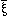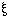HOME TOP UP PREV NEXT 1 2 3 GERMAN MAP      Tractatus Logico-Philosophicus 5.50

5.50

5.501
An expression in brackets whose terms are propositions I indicate -- if the order of the terms in the bracket is indifferent -- by a sign of the form "()". "" is a variable whose values are the terms of the expression in brackets, and the line over the veriable indicates that it stands for all its values in the bracket.

(Thus ifhas the 3 values P, Q, R, then () = (P, Q, R).)

The values of the variables must be determined.

The determination is the description of the propositions which the variable stands for.

How the description of the terms of the expression in brackets takes place is unessential.

We may distinguish 3 kinds of description: 1. Direct enumeration. In this case we can place simply its constant values instead of the variable. 2. Giving a function fx, whose values for all values of x are the propositions to be described. 3. Giving a formal law, according to which those propositions are constructed. In this case the terms of the expression in brackets are all the terms of a formal series.

5.502
Therefore I write instead of "(- - - - - T)(, . . . .)", "N()".

N() is the negation of all the values of the propositional variable.

5.503
As it is obviously easy to express how propositions can be constructioned by means of this operation and how propositions are not to be constructed by means of it, this must be capable of exact expression.

HOME TOP UP PREV NEXT 1 2 3 GERMAN MAP      Tractatus Logico-Philosophicus 5.50